# Ammeter Voltmeter method for measurement of medium resistance

## Ammeter Voltmeter method:

This is very popular method for measurement of medium resistances since instruments required for this method are usually available in laboratory. The two types of connections employed for ammeter voltmeter method are shown in figure. In both the methods if readings of ammeter and voltmeter are taken then we can measure value of resistance by using formula:The measured value of resistance Rm, would be equal to the true value R, if the ammeter resistance is zero and the voltmeter resistance is infinite, so that the conditions in the circuit are not disturbed. But in actual practice this is not possible and hence both methods give inaccurate results.

Consider circuit of figure (a):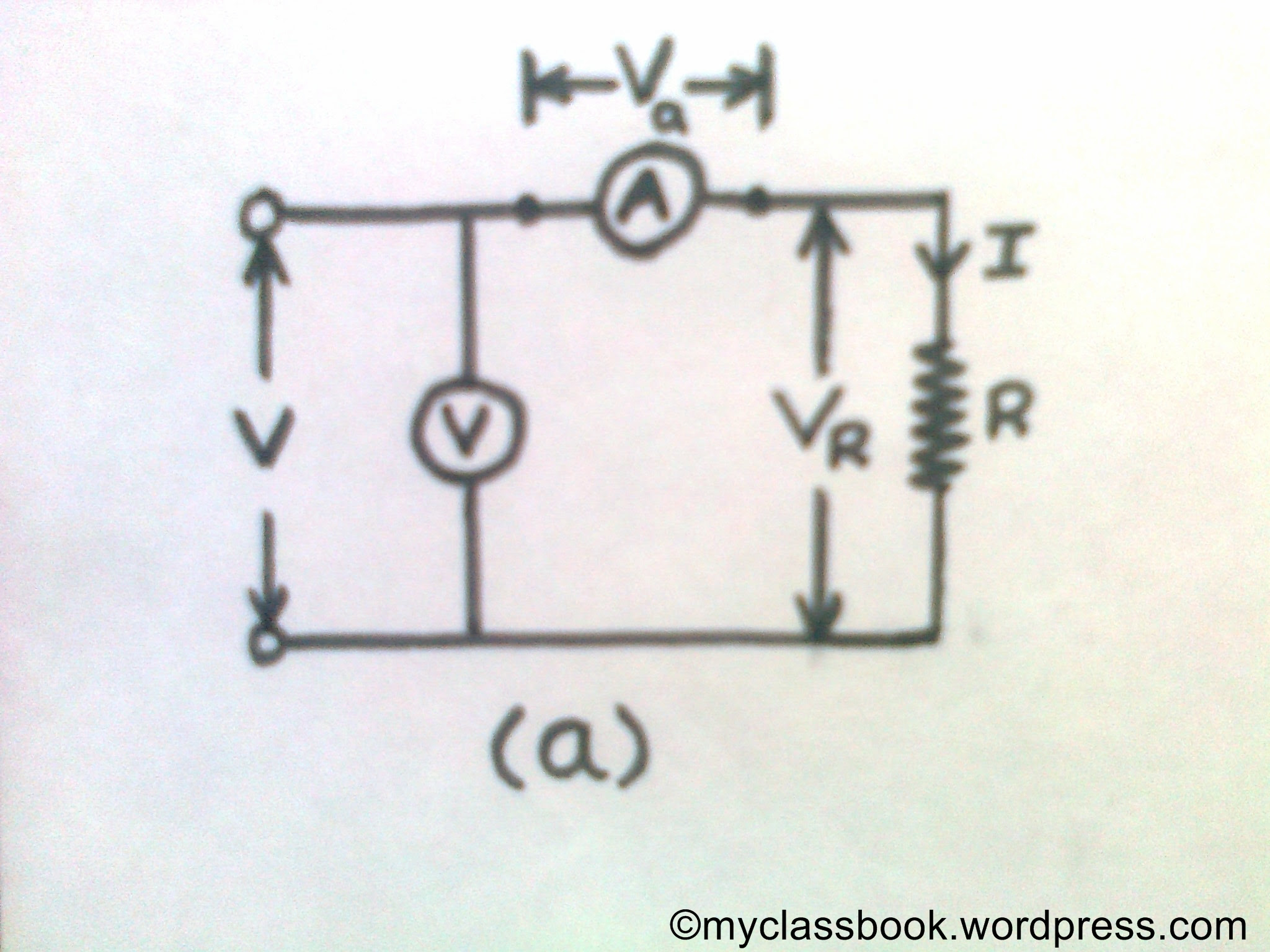voltmeter ammeter method

In this method ammeter measures the true value of current flowing through resistance but voltmeter does not measures the true value of the voltage across the resistance. the voltmeter indicates the sum of the voltage across resistance and ammeter.

Let Ra be the resistance of the ammeter.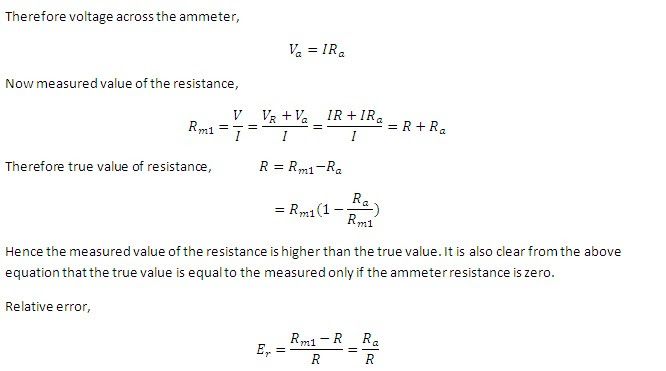It is clear from the above equation that the error will be small if the value of the measuring resistance is large as compare to the internal resistance of the ammeter .therefore circuit should be used when measuring resistances are high.

Consider circuit of figure (b):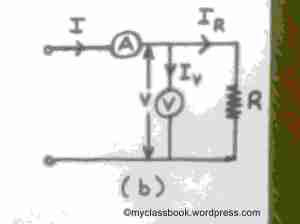voltmeter ammeter method

In this circuit the voltmeter measures the true value of the voltage across the measuring resistance but the ammeter does not measures the true value of the current flowing through the resistance .the current through the ammeter is the sum of the current through the voltmeter and resistance.

Let Rv be the resistance of the voltmeter.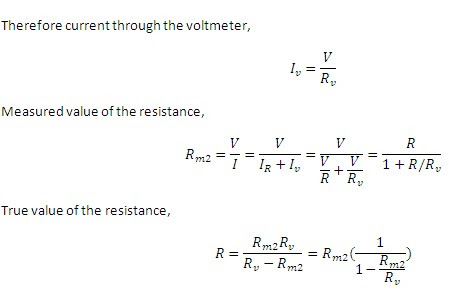From the above equation it is clear the true value of the resistance will be equal to the measured value only when the voltmeter resistance is equal to the infinite. However, if the resistance of the voltmeter is very large as compared to the resistance under measurement: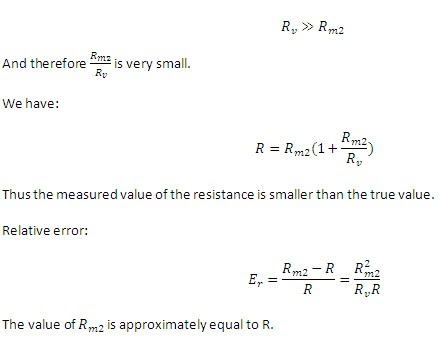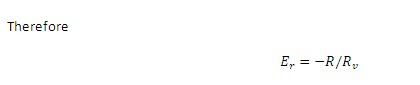It is clear from the above equation that the relative error will be small if the resistance under measurement is very small as compared to the resistance of the voltmeter .hence the circuit should be used when the measuring values of resistances are low.

1.